# Chapter 4 Fluid Description of Plasma

The single particle approach gets to be horribly complicated, as we have seen.
Basically we need a more statistical approach because we can't follow each particle separately. If the details of the distribution function in velocity space are important we have to stay with the Boltzmann equation. It is a kind of particle conservation equation.

## 4.1  Particle Conservation (In 3-d Space)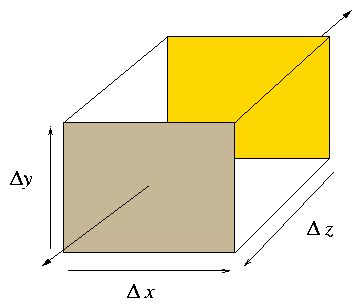Figure 4.1: Elementary volume for particle conservation
Number of particles in box ∆x ∆y ∆z is the volume, ∆V = ∆x ∆y ∆z, times the density n. Rate of change of number is is equal to the number flowing across the boundary per unit time, the flux. (In absence of sources.)
 − ∂ ∂t [∆x ∆y ∆z  n] =  Flow Out across boundary.
(4.1)
Take particle velocity to be v(r) [no random velocity, only flow] and origin at the center of the box refer to flux density as nv = J.
 Flow Out   =  [Jz ( 0, 0, ∆z/2 ) − Jz ( 0, 0, −∆z/2 ) ]  ∆x ∆y +  x  +  y   .
(4.2)
Expand as Taylor series
 Jz (0, 0, η) = Jz (0) + ∂ ∂z Jz  .  η
(4.3)
So,
 flow out
 ≅
 ∂ ∂z (n vz) ∆z ∆x∆y  +  x  + y
(4.4)
 =
 ∆V  ∇.(n v).
Hence Particle Conservation
 − ∂ ∂t n = ∇ . (n v)
(4.5)
Notice we have essential proved an elementary form of Gauss's theorem
 ⌠⌡ v ∇ . A d3 r   = ⌠⌡ ∂V A . dS .
(4.6)
The expression: `Fluid Description' refers to any simplified plasma treatment which does not keep track of v-dependence of f detail.
1. Fluid Descriptions are essentially 3-d (r).
2. Deal with quantities averaged over velocity space (e.g. density, mean velocity, ...).
3. Omit some important physical processes (but describe others).
4. Provide tractable approaches to many problems.
Fluid Equations can be derived mathematically by taking moments1 of the Boltzmann Equation.
 0th moment
 ⌠⌡
 d3 v
(4.7)
 1st  moment
 ⌠⌡
 vd3 v
(4.8)
 2nd  moment
 ⌠⌡
 vvd3 v
(4.9)
These lead, respectively, to (0) Particle (1) Momentum (2) Energy conservation equations.
We shall adopt a more direct `physical' approach.

## 4.2  Fluid Motion

The motion of a fluid is described by a vector velocity field v(r) (which is the mean velocity of all the individual particles which make up the fluid at r). Also the particle density n(r) is required. We are here discussing the motion of fluid of a single type of particle of mass/charge, m/q so the charge and mass density are qn and mn respectively.
The particle conservation equation we already know. It is also sometimes called the `Continuity Equation'
 ∂ ∂t n + ∇ . (n v) = 0
(4.10)
It is also possible to expand the ∇. to get:
 ∂ ∂t n + (v. ∇) n + n ∇ . v= 0
(4.11)
The significance, here, is that the first two terms are the `convective derivative" of n
 D Dt ≡ d dt ≡ ∂ ∂t + v. ∇
(4.12)
so the continuity equation can be written
 D Dt n = −n∇.v
(4.13)

### 4.2.1  Lagrangian & Eulerian Viewpoints

There are essentially 2 views.
1. Lagrangian. Sit on a fluid element and move with it as fluid moves.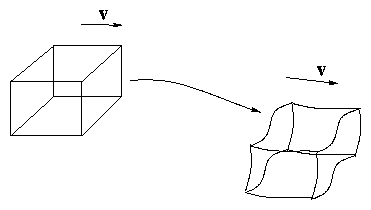Figure 4.2: Lagrangean Viewpoint
2. Eulerian. Sit at a fixed point in space and watch fluid move through your volume element: "identity" of fluid in volume continually changing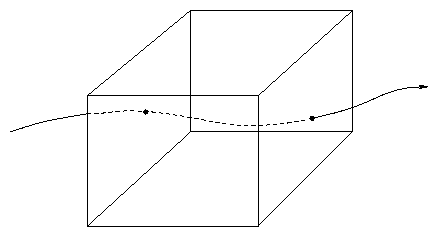Figure 4.3: Eulerian Viewpoint
[(∂)/(∂t)] means rate of change at fixed point (Euler).
[ D/Dt] ≡ [d/dt] ≡ [( ∂)/(∂t )] + v. ∇ means rate of change at moving point (Lagrange).
v.∇ = [dx/(∂t)] [(∂)/(∂x)]+[dy/(∂t)] [(∂)/(∂y)]+[dz/(∂t)] [(∂)/(∂z)] : change due to motion.
Our derivation of continuity was Eulerian. From the Lagrangian view
 D Dt n = d dt ∆N ∆V = − ∆N ∆V2 d dt ∆V = − n 1 ∆V d ∆V dt
(4.14)
since total number of particles in volume element (∆N) is constant (we are moving with them). (∆V = ∆x ∆y ∆z.)
 Now d dt ∆V
 =
 d∆x dt ∆y ∆z + d ∆y dt ∆z ∆x + d ∆z dt ∆y ∆x
(4.15)
 =
 ∆V ⎡⎣ 1 ∆x d ∆x dt + 1 ∆y d ∆y dt + 1 ∆x d ∆z dt ⎤⎦
(4.16)

 But d ( ∆x ) dt
 =
 vx ( ∆x / 2 ) − vx ( − ∆x / 2 )
(4.17)
 ≅
 ∆x ∂vx ∂x etc.     ... y     ... z
(4.18)
Hence
 d dt ∆V = ∆V ⎡⎣ ∂vx ∂x + ∂vy ∂y + ∂vz ∂z ⎤⎦ = ∆V ∇ . v
(4.19)
and so
 D Dt n = − n ∇. v
(4.20)
Lagrangian Continuity. Naturally, this is the same equation as Eulerian when one puts [D/Dt] = [(∂)/(∂t)] + v. ∇.
The quantity − ∇ . v is the rate of (Volume) compression of element.

### 4.2.2  Momentum (Conservation) Equation

Each of the particles is acted on by the Lorentz force q [E+ uiB] (ui is individual particle's velocity).
Hence total force on the fluid element due to E-M fields is
 ∑ i ( q [ E+ ui ∧B ] ) = ∆N  q ( E+ v∧B)
(4.21)
(Using mean: v = ∑i ui / ∆N.)
E-M Force density (per unit volume) is:
 FEM = n q (E+ v∧B).
(4.22)
The total momentum of the element is
 ∑ i m ui = m  ∆N  v = ∆V  mn v
(4.23)
so Momentum Density is mnv.
If no other forces are acting then clearly the equation of motion requires us to set the time derivative of mnv equal to FEM. Because we want to retain the identity of the particles under consideration we want D/Dt i.e. the convective derivative (Lagrangian picture).
In general there are additional forces acting.
(1) Pressure         (2) Collisional Friction.

### 4.2.3  Pressure Force

In a gas p( = nT) is the force per unit area arising from thermal motions. The surrounding fluid exerts this force on the element: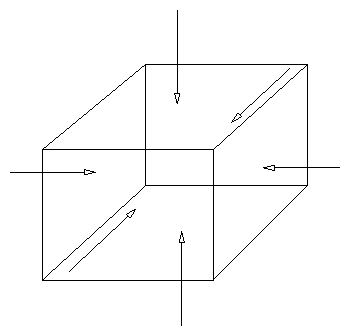Figure 4.4: Pressure forces on opposite faces of element.
Net force in x direction is
 −
 p ( ∆x / 2 ) ∆y ∆z + p ( − ∆x / 2 ) ∆y ∆z
(4.24)
 ≅
 − ∆x ∆y ∆z ∂p ∂x = − ∆V ∂p ∂x = − ∆V ( ∇p )x
(4.25)
So (isotropic) pressure force density (/unit vol)
 Fp = − ∇ p
(4.26)
How does this arise in our picture above?
Answer: Exchange of momentum by particle thermal motion across the element boundary.
Although in Lagrangian picture we move with the element (as defined by mean velocity v) individual particles also have thermal velocity so that the additional velocity they have is
 wi = ui − v          `peculiar′velocity
(4.27)
Because of this, some cross the element boundary and exchange momentum with outside. (Even though there is no net change of number of particles in element.) Rate of exchange of momentum due to particles with peculiar velocity w, d3w across a surface element ds is
 f( w ) m w  d3 wmomentum density at w × w  .  dsflow rate across ds
(4.28)
Integrate over distrib function to obtain the total momentum exchange rate:
 ds . ⌠⌡ m w w f (w) d3 w
(4.29)
The thing in the integral is a tensor. Write
 p   = ⌠⌡ m w w f (w) d3w           (Pressure Tensor)
(4.30)
Then momentum exchange rate is
 p  .  ds
(4.31)
Actually, if f(w) is isotropic (e.g. Maxwellian) then
 pxy
 =
 ⌠⌡ m  wx  wy  f (w) d3w = 0     etc.
(4.32)
 and  pxx
 =
 ⌠⌡ m wx2  f (w) d3w ≡ n T ( = pyy = pzz = `p′)
(4.33)
So then the exchange rate is pds. (Scalar Pressure).
Integrate ds over the whole ∆V then x component of momm exchange rate is
 p ⎛⎝ ∆x 2 ⎞⎠ ∆y ∆z− p ⎛⎝ − ∆x 2 ⎞⎠ ∆y ∆z = ∆V ( ∇ p )x
(4.34)
and so
Total momentum loss rate due to exchange across the boundary per unit volume is
 ∇ p        ( = − Fp )
(4.35)
In terms of the momentum equation, either we put ∇p on the momentum derivative side or Fp on force side. The result is the same.
Ignoring Collisions, Momentum Equation is
 D Dt ( m n ∆V v) = [FEM + Fp ] ∆V
(4.36)
Recall that n∆V = ∆N   ;    [D/Dt] (∆N) = 0; so
 D Dt ( m n ∆V v) = m n ∆V Dv dt .
(4.37)
Thus, substituting for F′s:
Momentum Equation.
 m n Dv Dt = m n ⎛⎝ ∂v ∂t + v. ∇ v ⎞⎠ = q n ( E + v∧B) − ∇p
(4.38)

### 4.2.4  Momentum Equation: Eulerian Viewpoint

Fixed element in space. Plasma flows through it.
1. E.M. force on element (per unit vol.)
 FEM = nq ( E+ v∧B)         as before.
(4.39)
2. Momentum flux across boundary (per unit vol)
 =
 ∇. ⌠⌡ m (v+ w) (v+ w)  f(w)  d3w
(4.40)
 =
 ∇. { ⌠⌡ m (vv+ vw + wvintegrates to 0 + w w)  f(w)  d3 w }
(4.41)
 =
 ∇. { m n vv+ p }
(4.42)
 =
 m n (v. ∇) v+ m v[ ∇ . (n v) ] + ∇p
(4.43)
(Take isotropic p.)
3. Rate of change of momentum within element (per unit vol)
 = ∂ ∂t ( m n v)
(4.44)
Hence, total momentum balance:
 ∂ ∂t (m n v) + m n (v. ∇) v+ m v[ ∇ . (n v) ] + ∇p = FEM
(4.45)
Use the continuity equation:
 ∂n ∂t + ∇ . (n v) = 0    ,
(4.46)
to cancel the third term and part of the 1st:
 ∂ ∂t (m n v) + m v( ∇ . ( n v) ) = m v{ ∂n ∂t + ∇ . ( n v) } + m n ∂v ∂t = m n ∂v ∂t
(4.47)
Then take ∇p to RHS to get final form:
Momentum Equation:
 m n ⎡⎣ ∂v ∂t + ( v. ∇ ) v ⎤⎦ = nq ( E+ v∧B) − ∇p  .
(4.48)
As before, via Lagrangian formulation. (Collisions have been ignored.)

### 4.2.5  Effect of Collisions

First notice that like particle collisions do not change the total momentum (which is averaged over all particles of that species).
Collisions between unlike particles do exchange momentum between the species. Therefore once we realize that any quasi-neutral plasma consists of at least two different species (electrons and ions) and hence two different interpenetrating fluids we may need to account for another momentum loss (gain) term.
The rate of momentum density loss by species 1 colliding with species 2 is:
 ν12 n1 m1 (v1 − v2)
(4.49)
Hence we can immediately generalize the momentum equation to
 m1 n1 ⎡⎣ ∂v1 ∂t + ( v1 . ∇ ) v1 ⎤⎦ = n1 q1 ( E+ v1 ∧B) − ∇p1 − ν12 n1 m1 ( v1 − v2 )
(4.50)
With similar equation for species 2.

## 4.3  The Key Question for Momentum Equation:

What do we take for p?
Basically p = nT is determined by energy balance, which will tell how T varies. We could write an energy equation in the same way as momentum. However, this would then contain a term for heat flux, which would be unknown. In general, the kth moment equation contains a term which is a (k + 1)th moment.
Continuity,  0th  equation contains v determined by
Momentum, 1st   equation contains p determined by
Energy,       2nd  equation contains Q determined by ...
In order to get a sensible result we have to truncate this hierarchy. Do this by some sort of assumption about the heat flux. This will lead to an
Equation of State:
 pn−γ = const.
(4.51)
The value of γ to be taken depends on the heat flux assumption and on the isotropy (or otherwise) of the energy distribution.
Examples
1. Isothermal: T = const.:      γ = 1.
2. Adiabatic/Isotropic: 3 degrees of freedom   γ = 5/3.
3. Adiabatic/1 degree of freedom    γ = 3.
4. Adiabatic/2 degrees of freedom    γ = 2.
In general, n (l/ 2) δT = − p (δV/V)     (Adiabatic l degrees)
 l 2 δT T = − δV V
 =
 + δn n
(4.52)
So
 δp p
 =
 δn n + δT T = ⎛⎝ 1 + 2 l ⎞⎠ δn n ,
(4.53)
i.e.
 p n −( 1 + [2/(l)]) = const.
(4.54)
In a normal gas, which `holds together' by collisions, energy is rapidly shared between 3 space-degrees of freedom. Plasmas are often rather collisionless so compression in 1 dimension often stays confined to 1-degree of freedom. Sometimes heat transport is so rapid that the isothermal approach is valid. It depends on the exact situation; so let's leave γ undefined for now.

## 4.4  Summary of Two-Fluid Equations

Species j
Plasma Response
1. Continuity:
 ∂nj ∂t + ∇ . (njvj) = 0
(4.55)
2. Momentum:
 mjnj ⎡⎣ ∂vj ∂t + ( vj . ∇ ) vj ⎤⎦ = njqj ( E+ vj ∧B) − ∇pj − - ν jk njmj (vj − vk )
(4.56)
3. Energy/Equation of State:
 pj nj−γ = const..
(4.57)
(j = electrons, ions).
Maxwell's Equations
 ∇ . B
 =
 0                      ∇ . E = ρ/ϵo
(4.58)
 ∇ ∧B
 =
 μo j + 1 c2 ∂E ∂t ∇ ∧E = −∂B ∂t
(4.59)
With
 ρ
 =
 qe ne + qi ni = e ( −ne + Zni )
(4.60)
 j
 =
 qe ne ve + qi ni vi = e ( −ne ve + Z ni vi )
(4.61)
 =
 −e ne ( ve − vi r)      (Quasineutral)
(4.62)
Accounting
 Unknowns Equations ne,ni 2 Continuity e, i 2 ve,vi 6 Momentum e,i 6 pe,pi 2 State e,i 2 E, B 6 Maxwell 8 16 18
but 2 of Maxwell (∇. equs) are redundant because can be deduced from others: e.g.
 ∇ . ( ∇ ∧E)
 =
 0 = − ∂ ∂t ( ∇ . B)
(4.63)
 and  ∇ . ( ∇ ∧B)
 =
 0 = μo ∇ . j + 1 c2 ∂ ∂t ( ∇ . E) = 1 c2 ∂ ∂t ⎛⎝ −ρ ϵo + ∇ . E ⎞⎠
(4.64)
So 16 equs for 16 unknowns.
Equations still very difficult and complicated mostly because it is Nonlinear
In some cases can get a tractable problem by `linearizing'. That means, take some known equilibrium solution and suppose the deviation (perturbation) from it is small so we can retain only the 1st linear terms and not the others.

## 4.5  Two-Fluid Equilibrium: Diamagnetic Current

Slab:          [(∂)/(∂x)] ≠ 0      [(∂)/(∂y)], [(∂)/(∂z)] = 0.
Straight B-field:    B = Bz.
Equilibrium:          [(∂)/(∂t)] = 0        (E = − ∇ϕ)
Collisionless:      ν→ 0.
Momentum Equation(s):
 mjnj (vj . ∇) vj = nj qj (E+ vj ∧B) − ∇pj
(4.65)
Drop j suffix for now. Then take x,y components:
 mn vx d dx vx
 =
 nq (Ex + vy B) − dp dx
(4.66)
 mn vx d dx vy
 =
 nq (0 −vx B)
(4.67)
Eq 4.67 is satisfied by taking vx = 0. Then 4.66
 nq (Ex + vy B) − dp dx = 0.
(4.68)
i.e.
 vy = − Ex B + 1 nqB dp dx
(4.69)
or, in vector form:
v=

 E∧B B2

EB drift

 ∇ p nq ∧ B B2

Diamagnetic Drift

(4.70)
Notice:
• In magnetic field (⊥) fluid velocity is determined by component of momentum equation orthogonal to it (and to B).
• Additional drift (diamagnetic) arises in standard FB form from pressure force.
• Diagmagnetic drift is opposite for opposite signs of charge (electrons vs. ions).
Now restore species distinctions and consider electrons plus single ion species i. Quasineutrality says niqi = −neqe. Hence adding solutions
 neqeve + niqivi = E∧B B2 ( niqi + neqe )=0 − ∇( pe + pi )∧ B B2
(4.71)
Hence current density:
 j = − ∇( pe + pi ) ∧ B B2
(4.72)
This is the diamagnetic current. The electric field, E, disappears because of quasineutrality. (General case ∑j qjnjvj = − ∇(∑pj) ∧B/ B2).

## 4.6  Two-Fluid Dynamics

We shall see that two-fluid treatments are widely used the question of waves, but before we get into that in detail, let us look briefly at some time-varying situations for two-fluid treatments.

### 4.6.1  Parallel Two-Fluid Dynamics

When motion is in the parallel-to-B direction, the fluid momentum equation becomes
 m n ⎡⎣ ∂ ∂t + v ∂ ∂ z ⎤⎦ v = nq E − ∂p ∂z ,
(4.73)
where z is the parallel coordinate, all components are parallel, and perpendicular variation is taken to be absent.
An equation like this applies to both the electrons and ions. Now consider a one-dimensional compression of the plasma so that the density varies with z. Start with electrons. They are very light compared with ions, so if the time derivative is relatively slow, the rate of change of their momentum, which is the left hand side of this equation, is negligible. Also, since their rapid thermal conduction along the field lines will oppose any temperature variation let us suppose that the electron temperature Te is uniform. (That's our equation of state for electrons). In that case as an approximation we can write
 −qe ∂ϕ ∂z =qe E = 1 ne ∂ p ∂z = Te ne ∂ ne ∂z = Te ∂ln(ne) ∂z .
(4.74)
This equation can simply be integrated from some arbitrary reference point where ϕ = 0 and ne=ne0 to find ln(ne/ne0) = −qeϕ/Te or
 ne = ne0 exp( eϕ Te ).
(4.75)
This is the Boltzmann factor that we invoked for a thermal equilibrium situation at the beginning of the course. We see that the fluid equations derive the same variation along the magnetic field, assuming the electrons to be in equilibrium. The pressure gradient term and the electric force balance each other.
Now what about the ions? We cannot ignore their inertia because they are heavy. But let us suppose that they have only a very small velocity so that the second-order convective derivative term can be ignored, and only mini∂vi/∂t remains on the left hand side of eq. (4.73). Using our solution for the relationship between ϕ and ne, which implies ∂ϕ/∂z = (e/ne)∂ne/∂z we can then write the ion momentum equation as
 mini ∂vi ∂t =niqiE − ∂ pi ∂z = niqi neqe Te ∂ne ∂ z − ∂pi ∂z
(4.76)
We now recognize the effect of quasi-neutrality is that there can be very little difference between −qene and qini, so we set them equal (this is sometimes called the "plasma approximation"). Also, if the ion equation of state is pi niγi = constant, then we can write
 ∂pi ∂z = γi pi ni ∂ ni ∂z = γiTi ∂ni ∂z
(4.77)
and the momentum equation becomes
 ρi ∂vi ∂t = −(Z Te+γiTi) ∂ ni ∂z ,
(4.78)
where Z=|qi/qe| is the ion charge-number and ρi=nimi is the ion mass density. This ion momentum equation is of just the form that a standard gas would have, except that instead of just γT we have ZTei Ti. It states that the ion fluid has a rate of change of momentum density equal to minus a pressure gradient. But the pressure gradient involves not just the ion pressure, it also involves the electron pressure. What is happening is that the electron pressure gradient is being sustained by the ion inertia because the electric field (through ϕ) transfers force between the electrons and the ions.
To complete the physics we need the continuity equation
 ∂ni ∂t = − ∂(ni vi) ∂ z ≅ − ni ∂vi ∂ z ,
(4.79)
(ignoring vi∂ni/∂z as second order assuming small variation of ni and small vi) which allows us to derive a combined partial differential equation for each perturbed quantity alone, for example eliminate vi and we get an equation for ni:
 ∂2 ni ∂t2 ≅ ⎡⎣ ZTe+γi Ti mi ⎤⎦ ∂2 ni ∂z2 ≡ cs2 ∂2 ni ∂z2 .
(4.80)
This can easily be recognized as a one dimensional wave equation whose speed of propagation (cs) squared is the square bracket. This is called the ion sound (or acoustic) speed.
Warning   This fluid treatment turns out to omit an important part (ion Landau damping) of the physics of longitudinal acoustic oscillations , as we will see later when we do a kinetic theory treatment. The fluid treatment is valid only when Ti << Te, in which case the sound speed is cs=√{ZTe/mi}, and only the electron pressure matters.

### 4.6.2  Drift Waves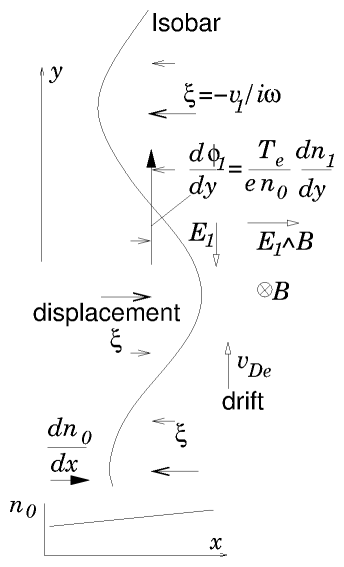Figure 4.5: Mechanism of driftwaves
A another type of wave that requires two-fluids for its existence depends upon a density gradient. Consider a configuration like that used to discuss the diamagnetic drifts, where there is a background density (n0) gradient only in the x-direction [(∂n)/(∂x)]=n0′ (temperatures are taken uniform for simplicity). Suppose there is a perturbative velocity v1 in the x-direction that is independent of x but varies sinusoidally in the y-direction and in time so v1 ∝ expi(ky−ωt) and similar small perturbations arise in the other parameters too: n1 and ϕ1. We will suppose that the magnetic field is almost entirely in the z-direction, except that there is a tiny but sufficient By component to allow us to use the electron parallel pressure balance equation to conclude that the electron density is governed by the Boltzmann factor n ∝ exp(eϕ/Te), but not enough By to affect anything else. For small perturbative displacements (dropping second order terms like n1v1) the continuity equation is
 ∂n1 ∂t = −iωn1=− ∂ ∂ x (nv) ≅ −v1 n0′.
(4.81)
The Boltzmann factor relates the value of n1 to ϕ1 from eϕ1/Te = n1/n0. But why would there be any v1 in the x-direction? Because there is a potential gradient in the y direction giving Ey=−[(∂ϕ1)/(∂y)]=ikϕ1, and consequently an E∧B drift
 v1= EyBz B2 =−ik ϕ1 B .
(4.82)
We therefore have a self-consistent solution when
 −ik ϕ1 B =v1 = iω n1 n0′ = iω eϕ1 Te n0 n0′ .
(4.83)
Cancelling ϕ1 from the first and last expression we find the "dispersion relation", which relates ω and k:
 ω k = − Te eB n0 ∂n0 ∂x =vDe.
(4.84)
This expression shows the phase velocity ω/k is exactly the diamagnetic drift velocity of the electrons. So we have found that there exist waves consisting of transverse displacement (velocity) perpendicular to B and k that propagate at the velocity of the electron diamagnetic drift. Notice that these waves have v1 exactly 90 degrees out of phase (multiplied by i) with respect to ϕ1 and n1. If some other physics (for example finite parallel resistivity) intervenes in addition giving rise to a change of this phase shift, these waves can become unstable.

## 4.7  Reduction of Fluid Approach to the Single Fluid Equations

So far we have been using fluid equations which apply to electrons and ions separately. These are called `Two Fluid' equations because we always have to keep track of both fluids separately.
A further simplification is possible and useful sometimes by combining the electron and ion equations together to obtain equations governing the plasma viewed as a `Single Fluid'.
Recall 2-fluid equations:
 Continuity  (Cj)
 ∂nj ∂t + ∇. (njvj) = 0 .
(4.85)
 Momentum  (Mj)
 mjnj ⎛⎝ ∂ ∂t + vj . ∇ ⎞⎠ vj = njqj ( E+ vj ∧B) − ∇pj + Fjk
(4.86)
(where we just write Fjk = −vjknjmj ( vjvk ) for short.)
Now we rearrange these 4 equations (2 × 2 species) by adding and subtracting appropriately to get new equations governing the new variables:

 Mass  Density               ρm
 =
 neme + nimi
(4.87)
 C of M Velocity            V
 =
 ( neme ve + nimivi ) / ρm
(4.88)
 Charge density              ρq
 =
 qene + qini
(4.89)
 Electric Current Density  j
 =
 qeneve +qinivi
(4.90)
 =
 qe ne ( ve−vi )  by quasi neutrality
(4.91)
 Total  Pressure                p
 =
 pe + pi
(4.92)
1st equation: take me ×Ce + mi ×Ci

 (1) ∂ρm ∂t + ∇ . ( ρm V ) = 0      Mass Conservation
(4.93)
2nd take qe ×Ce + qI ×Ci
 (2) ∂ρq ∂t + ∇ . j = 0          Charge Conservation
(4.94)
3rd take Me + Mi. This is a bit more difficult. RHS becomes:
 ∑ njqj ( E+ vj ∧B) − ∇pj + Fjk = ρq E+ j ∧B− ∇( pe + pi )
(4.95)
(we use the fact that Fei − Fie so no net friction). LHS is
 ∑ j mj nj ⎛⎝ ∂ ∂t + vj .∇ ⎞⎠ vj .
(4.96)
The difficulty here is that the convective term is non-linear and so does not easily lend itself to reexpression in terms of the new variables. But note that since me << mi the contribution from electron momentum is much less than that from ions and we can usually ignore it in this equation. Also to the same degree of approximation (dropping terms of order me/mi) Vvi: the CM velocity is the ion velocity. Thus for the LHS of this momentum equation we take
 ∑ j mini ⎛⎝ ∂ ∂t + vj . ∇ ⎞⎠ vj ≅ ρm ⎛⎝ ∂ ∂t + V . ∇ ⎞⎠ V,
(4.97)
so:
 (3)      ρm ⎛⎝ ∂ ∂t + V . ∇ ⎞⎠ V = ρq E+ j ∧B− ∇p.
(4.98)
Finally we take [(qe)/(me)] Me + [(qi)/(mi)] Mi to get:
 ∑ j nj qj ⎡⎣ ∂ ∂t + ( vj . ∇ ) ⎤⎦ vj = ∑ j { njqj2 mj ( E+ vj ∧B) − qj mj ∇pj + qj mj Fjk }.
(4.99)
Again difficulties arise with the nonlinear terms. Observe that the LHS can be written (using quasineutrality niqi + neqe = 0) as ρm [(∂)/(∂t)] ( [(j)/(ρm)]) plus the nonlinear term. Since ve=vi+j/neqe, the nonlinear term is
 ∑ j njqj(vj . ∇ ) vj = j.∇vi + vi.∇j + j.∇j/neqe
(4.100)
Compare the magnitude of these terms, neqevivd and neqevd2 (where vd=j/neqe is the relative drift velocity associated with current) with the magnitude of qe∇pe/me ∼ qe ne vte2 (on the RHS). In the usual case that both vi and vd are of order vti or less, the nonlinear term is smaller by of order me/mi, and so it can be dropped.
In the R.H.S. we use quasineutrality again to write
 ∑ j njqj2 mj E
 =
 ne2 qe2 ⎛⎝ 1 neme + 1 nimi ⎞⎠ E = ne2qe2 mini+mene nemenimi E = − qeqi memi ρm E,
(4.101)
 ∑ njqq2 mj vj
 =
 neqe2 me ve + niqi2 mi vi
 =
 qeqi memi { neqemi qi ve + niqime qe vi }
 =
 − qeqi memi { nemeve + nimivi − ⎛⎝ mi qi + me qe ⎞⎠ ( qeneve + qinivi ) }
 =
 − qeqi memi { ρm V − ⎛⎝ mi qi + me qe ⎞⎠ j }
(4.102)
Also, remembering Fei = −νei nemi (vevi) = − Fie,
 ∑ j qj mj Fjk
 =
 − ν ei ⎛⎝ neqe − neqi me mi ⎞⎠ ( ve − vi )
 =
 − ν ei ⎛⎝ 1 − qe qi me mi ⎞⎠ j
(4.103)
So we get
 ρm ∂ ∂t ⎛⎝ j ρm ⎞⎠
 =
 − qeqi memi ⎡⎣ ρm E+ ⎧⎨ ⎩ ρm V − ⎛⎝ mi qi + me qe ⎞⎠ j ⎫⎬ ⎭ ∧B ⎤⎦
 − qe me ∇pe − qi mi ∇pi − ⎛⎝ 1 − qe qi me mi ⎞⎠ ν ei j
(4.104)
Regroup after multiplying by [(memi)/(qeqiρm)]:
 E+ V ∧B
 =
 − memi qeqi ∂ ∂t ⎛⎝ j ρm ⎞⎠ + 1 ρm ⎛⎝ mi qi + me qe ⎞⎠ j ∧B
(4.105)
 − ⎛⎝ qe me ∇pe + qi mi ∇pi ⎞⎠ memi ρmqeqi − ⎛⎝ 1 − qe qi me mi ⎞⎠ memi qeqiρm ν ei j
Notice that this is an equation relating the Electric field in the frame moving with the fluid (L.H.S.) to things depending on current j i.e. this is a generalized form of Ohm's Law.
One essentially never deals with this full generalized Ohm's law. Make some approximations recognizing the physical significance of the various R.H.S. terms.
 memi qeqi ∂ ∂t ⎛⎝ j ρm ⎞⎠ arises from electron inertia.
It will be negligible for low enough frequency.
 1 ρm ⎛⎝ mi qi + me qe ⎞⎠ j ∧B is called the  Hall Term
and arises because current flow in a B-field tends to be diverted across the magnetic field. The me/qe term in it can be dropped. The whole Hall term is also often dropped but that is not generally well justified by ordering. [It can be justified sometimes by the fact that it does not appear in the component parallel to B.]
The ion pressure gradient can safely be ignored because
 qi mi ∇pi << qe me ∇pe
for comparable pressures. The electron pressure term is  ∼  the Hall term.
The last term in j has a coefficient, ignoring me/ mi c.f. 1 which is
 memi ν ei

qeqi ( nimi )
=
 me ν ei

qe2 ne
= η   the resistivity.
(4.106)
Hence dropping electron inertia, Hall term and pressure, the simplified Ohm's law becomes:
 E+ V ∧B= ηj .
(4.107)
Final equation needed: state:
 pene−γe + pini−γi = constant.
Take quasi-neutrality ⇒ ne ∝ ni ∝ ρm. Take γe = γi, then
 p ρm−γ = const.
(4.108)

### 4.7.1  Summary of Single Fluid Equations: M.H.D.

 Mass Conservation: ∂ρm ∂t + ∇( ρm V ) = 0
(4.109)

 Charge  Conservation: ∂ρq ∂t + ∇. j = 0
(4.110)

 Momentum:       ρm ⎛⎝ ∂ ∂t + V . ∇ ⎞⎠ V = ρq E+ j ∧B− ∇p
(4.111)

 Ohm′s  Law:                  E+ V ∧B = ηj
(4.112)

 Eq.  of  State:                 p ρm−γ = const.
(4.113)

### 4.7.2  Heuristic Derivation/Explanation

Mass Charge: Obvious.

Momm

 ρm ⎛⎝ ∂ ∂t + V . ∇ ⎞⎠ V

[(rate of change of) || (total momentum density)]

=

ρq E
[(Electric) || (body force)]
+

jB
[(Magnetic Force) || (on current)]

∇p
Pressure
(4.114)
Ohm's Law
The electric field `seen' by a moving (conducting) fluid is E+ VB = EV electric field in frame in which fluid is at rest. This is equal to `resistive' electric field ηj:
 EV = E+ V ∧B= ηj
(4.115)
The ρqE term is generally dropped because it is much smaller than the jB term. To see this, take orders of magnitude:
 ∇.E= ρq/ϵ0     so    ρq  ∼ Eϵ0/L
(4.116)

 ∇∧B=μ0j ⎛⎝ + 1 c2 ∂E ∂t ⎞⎠ so     σE = j  ∼ B/μ0 L
(4.117)
Therefore
 ρqE jB ∼ ϵ0 L ⎛⎝ B μ0σL ⎞⎠ 2 Lμ0 B2 ∼ L2/ c2 (μ0σL2)2 = ⎛⎝ light transit time resistive skin time ⎞⎠ 2 .
(4.118)
This is generally a very small number. For example, even for a small cold plasma, say Te=1 eV (σ ≈ 2×103 mho/m), L=1 cm, this ratio is about 10−8.
Conclusion: the ρq E force is much smaller than the jB force for essentially all practical cases. Ignore it.
Normally, also, one uses MHD only for low frequency phenomena, so the Maxwell displacement current, ∂E/c2∂t can be ignored.
Also we shall not need Poisson's equation because that is taken care of by quasi-neutrality.

### 4.7.3  Maxwell's Equations for MHD Use

 ∇ . B= 0   ;   ∇ ∧E = − ∂B ∂t ;   ∇ ∧B = μoj   .
(4.119)
The MHD equations find their major use in studying macroscopic magnetic confinement problems. In Fusion we want somehow to confine the plasma pressure away from the walls of the chamber, using the magnetic field. In studying such problems MHD is the major tool.
On the other hand if we focus on a small section of the plasma as we do when studying short-wavelength waves, other techniques: 2-fluid or kinetic are needed. Also, plasma is approx. uniform.
`Macroscopic' Phenomena MHD
`Microscopic' Phenomena 2-Fluid/Kinetic

## 4.8  MHD Equilibria

Study of how plasma can be `held' by magnetic field. Equilibrium ⇒ V = [(∂)/(∂t)] = 0. So equations reduce. Continuity and Faraday's law are  ∼  automatic. We are left with
 (Momm) → `Force Balance′    0 = j ∧B− ∇ p
(4.120)

 Ampere        ∇ ∧B = μo j
(4.121)
Plus ∇ . B = 0, ∇ . j = 0.
Notice that provided we don't ask questions about Ohm's law. E doesn't come into MHD equilibrium.
These deceptively simple looking equations are the subject of much of Fusion research. The hard part is taking into account complicated geometries.
We can do some useful calculations on simple geometries.

### 4.8.1  θ-pinch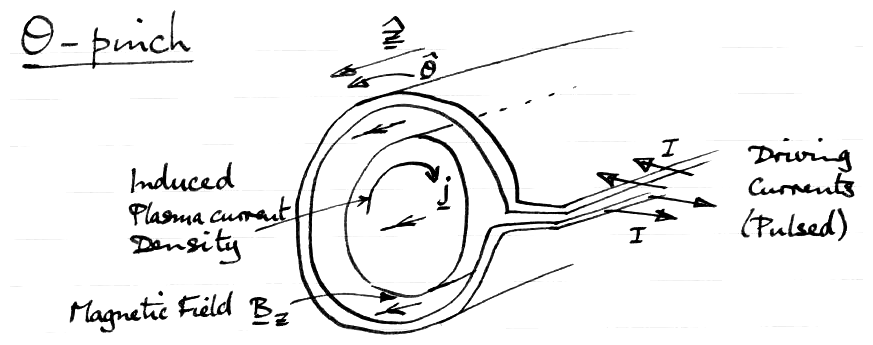Figure 4.6: θ-pinch configuration.
So called because plasma currents flow in θ-direction.
Use MHD Equations
Take to be ∞ length, uniform in z-dir.
By symmetry B has only z component.
By symmetry j has only θ- comp.
By symmetry ∇p has only r comp.
So we only need

 Force      ( j ∧B)r
 −
 ( ∇p )r = 0
(4.122)
 Ampere     ( ∇ ∧B)θ
 =
 ( μo j )θ
(4.123)
 i.e.      jθ Bz
 −
 ∂ ∂r p = 0
(4.124)
 − ∂ ∂r Bz
 =
 μo jθ
(4.125)
 Eliminate  j:    − Bz μo ∂Bz ∂r − ∂p ∂r
 =
 0
(4.126)
i.e.
 ∂ ∂r ⎛⎝ Bz2 2 μo + p ⎞⎠
 =
 0
(4.127)
 Solution Bz2 2 μo + p
 =
 const.
(4.128)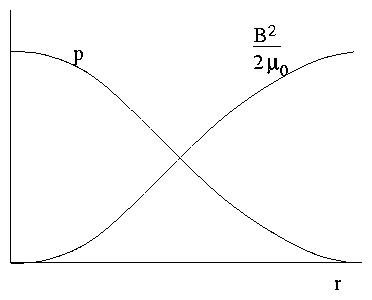Figure 4.7: Balance of kinetic and magnetic pressure

 Bz2 2 μo + p = Bz ext2 2 μo
(4.129)
[Recall Single Particle Problem]
Think of these as a pressure equation. Equilibrium says total pressure = const.

 Bz2 2 μo

magnetic pressure

+

p
kinetic pressure
= const.
(4.130)
Ratio of kinetic to magnetic pressure is plasma `β'.
 β = 2 μo p Bz2
(4.131)
measures `efficiency' of plasma confinement by B. Want large β for fusion but limited by instabilities, etc.

### 4.8.2  Z-pinch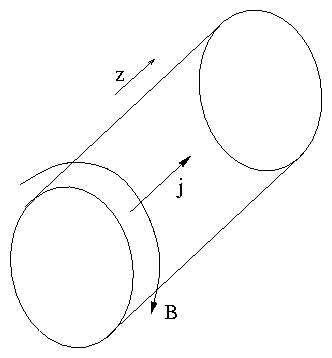Figure 4.8: Z-pinch configuration.
so called because j flows in z-direction. Again take to be ∞ length and uniform.
 j = jz ^ e z B = Bθ ^ e θ
(4.132)

 Force    ( j ∧B)r − ( ∇p )r = − jz Bθ − ∂p ∂r = 0
(4.133)

 Ampere    ( ∇ ∧B)z − ( μo j )z = 1 r ∂ ∂r ( r Bθ ) − μojz = 0
(4.134)
Eliminate j:
 Bθ μo r ∂ ∂r ( r Bθ ) + ∂p ∂r = 0
(4.135)
or

 Bθ2 μo r

Extra Term

+

∂r

 ⎛⎝ Bθ2 2 μo +       p ⎞⎠

Magnetic + Kinetic pressure

= 0
(4.136)
Extra term acts like a magnetic tension force. Arises because B-field lines are curved.
Can integrate equation
 ⌠⌡ b a Bθ2 μo dr r + ⎡⎣ Bθ2 2 μo + p(r) ⎤⎦ b a = 0
(4.137)
If we choose b to be edge (p(b)=0) and set a=r we get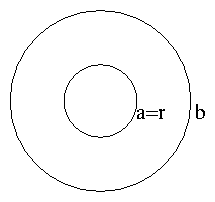Figure 4.9: Radii of integration limits.

 p(r) = Bθ2 ( b ) 2 μo − Bθ2 ( r ) 2 μo + ⌠⌡ b r Bθ2 μo dr′ r′
(4.138)
Force balance in z-pinch is somewhat more complicated because of the tension force. We can't choose p(r) and j(r) independently; they have to be self consistent.
Example    j = const.
 1 r ∂ ∂r ( r Bθ ) = μo jz ⇒ Bθ = μo jz 2 r
(4.139)
Hence
 p(r)
 =
 1 2 μo ⎛⎝ μo jz 2 ⎞⎠ 2 { b2 − r2 + ⌠⌡ b r 2 r′dr′}
(4.140)
 =
 μo jz2 4 { b2 − r2 }
(4.141)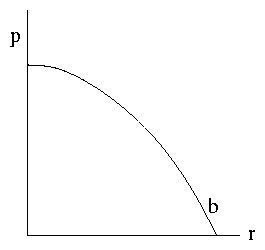Figure 4.10: Parabolic Pressure Profile.
Also note Bθ (b) = [( μo jzb)/2] so
 p = Bθb2 2 μo 2 b2 { b2 − r2 }
(4.142)

### 4.8.3  `Stabilized Z-pinch'

Also called `screw pinch', θ−z pinch or sometimes loosely just `z-pinch'.
Z-pinch with some additional Bz as well as Bθ
 (Force)r     jθ Bz − jz Bθ − ∂p ∂r = 0
(4.143)

 Ampere: ∂ ∂r Bz = μojθ
(4.144)

 1 r ∂ ∂r ( r Bθ ) = μo jz
(4.145)
Eliminate j:

 − Bz μo ∂Bz ∂r − Bθ μo r ∂ ∂r ( r Bθ ) − ∂p ∂r = 0
(4.146)
or

 Bθ2 μo r

[(Mag Tension) || (θ only)]

+

∂r

 ⎛⎝ B2 2 μo +        p ⎞⎠

Mag (θ+z) + Kinetic pressure

= 0
(4.147)

## 4.9  Some General Properties of MHD Equilibria

### 4.9.1  Pressure & Tension

 j ∧B− ∇p = 0    :    ∇ ∧B = μo j
(4.148)
We can eliminate j in the general case to get
 1 μo ( ∇ ∧B) ∧B= ∇p .
(4.149)
Expand the vector triple product:
 ∇p = 1 μo ( B. ∇ )B− 1 2 μo ∇ B2
(4.150)
put b = [(B)/ | B | ] so that ∇B = ∇(B b) = B ∇b + b ∇B. Then
 ∇p
 =
 1 μo { B2 ( b . ∇ ) b + B b ( b . ∇ ) B } − 1 2 μo ∇ B2
(4.151)
 =
 B2 μo ( b . ∇ )b − 1 2 μo ( ∇ − b ( b . ∇ ) ) B2
(4.152)
 =
 B2 μo ( b . ∇ ) b − ∇⊥ ⎛⎝ B2 2 μ0 ⎞⎠
(4.153)
Now ∇ ( [(B2)/(2 μo)] ) is the perpendicular (to B) derivative of magnetic pressure and (b . ∇)b is the curvature of the magnetic field line giving tension.
| ( b .∇ ) b | has value 1/R. R: radius of curvature.

### 4.9.2  Magnetic Surfaces

 0 = B. [ j ∧B− ∇p ] = − B. ∇ p
(4.154)
*Pressure is constant on a field line (in MHD situation).
(Similarly, 0 = j . [ jB− ∇p ] = j . ∇ p.)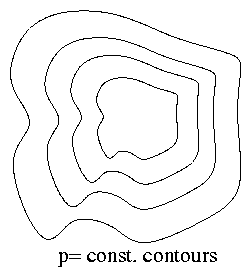Figure 4.11: Contours of pressure.
Consider some arbitrary volume in which ∇p ≠ 0. That is, some plasma of whatever shape. Draw contours (surfaces in 3-d) on which p = const. At any point on such an isobaric surface ∇p is perp to the surface. But B. ∇ p = 0 implies that B is also perp to ∇p.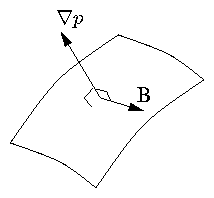Figure 4.12: B is perpendicular to ∇p and so lies in the isobaric surface.
Hence
B lies in the surface p = const.
In equilibrium isobaric surfaces are `magnetic surfaces'.
[This argument does not work if p = const. i.e. ∇ p = 0. Then there need be no magnetic surfaces.]

### 4.9.3  `Current Surfaces'

Since j . ∇ p = 0 in equilibrium the same argument applies to current density. That is
j lies in the surface p = const.
Isobaric Surfaces are `Current Surfaces'.
Moreover it is clear that
`Magnetic Surfaces' are `Current Surfaces'.
(since both coincide with isobaric surfaces.)
[It is important to note that the existence of magnetic surfaces is guaranteed only in the MHD approximation when ∇p ≠ 0 Taking account of corrections to MHD we may not have magnetic surfaces even if ∇p ≠ 0.]

### 4.9.4  Low β equilibria: Force-Free Plasmas

In many cases the ratio of kinetic to magnetic pressure is small, β << 1 and we can approximately ignore ∇p. Such an equilibrium is called `force free'.
 j ∧B= 0
(4.155)
implies j and B are parallel.
i.e.
 j = μ(r) B
(4.156)
Current flows along field lines not across. Take divergence:
 0
 =
 ∇ . j = ∇ . ( μ( r ) B) = μ( r ) ∇ . B+ ( B. ∇ ) μ
(4.157)
 =
 ( B. ∇ ) μ.
(4.158)
The ratio j/B = μ is constant along field lines.
μ is constant on a magnetic surface. If there are no surfaces, μ is constant everywhere.

#### Example: Force-Free Cylindrical Equil.

 j ∧B
 =
 0    ⇔    j = μ(r) B
(4.159)
 ∇ ∧B
 =
 μo j = μo μ(r) B
(4.160)
This is a somewhat more convenient form because it is linear in B (for specified μ(r)).
 Constant−μ:      ∇ ∧B = μo μB
(4.161)
leads to a Bessel function solution

 Bz
 =
 Bo J0 (μo μr)
(4.162)
 Bθ
 =
 Bo J1 (μo μr)
(4.163)
for μo μr > 1st zero of J0 the toroidal field reverses. There are plasma confinement schemes with μ ≅ const. `Reversed Field Pinch'.

## 4.10  Toroidal Equilibrium

Bend a z-pinch into a torus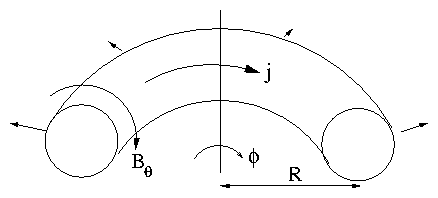Figure 4.13: Toroidal z-pinch
Bθ fields due to current are stronger at small R side ⇒ Pressure (Magnetic) Force outwards.
Have to balance this by applying a vertical field Bv to push plasma back by jϕBv.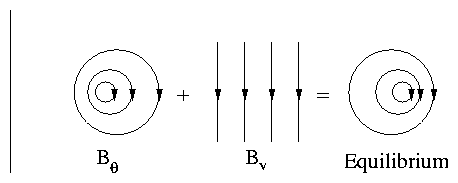Figure 4.14: The field of a toroidal loop is not an MHD equilibrium. Need to add a vertical field.
Bend a θ-pinch into a torus: Bϕ is stronger at small R side ⇒ outward force.
Cannot be balanced by Bv because no jϕ. No equilibrium for a toroidally symmetric θ-pinch.
Underlying Single Particle reason:
Toroidal θ-pinch has Bϕ only. As we have seen before, curvature drifts are uncompensated in such a configuration and lead to rapid outward motion.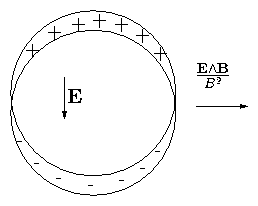Figure 4.15: Charge-separation giving outward drift is equivalent to the lack of MHD toroidal force balance.
We know how to solve this: Rotational Transform: get some Bθ. Easiest way: add jϕ. From MHD viewpoint this allows you to push the plasma back by jϕBv force. Essentially, this is Tokamak.

## 4.11  Plasma Dynamics (MHD)

When we want to analyze non-equilibrium situations we must retain the momentum terms. This will give a dynamic problem. Before doing this, though, let us analyse some purely Kinematic Effects.
`Ideal MHD'     ⇔ Set η = 0 in Ohm's Law.
A good approximation for high frequencies, i.e. times shorter than resistive decay time.
 E+ V ∧B= 0 .         Ideal  Ohm′s  Law.
(4.164)
Also
 ∇ ∧E= − ∂B ∂t Faraday′s  Law.
(4.165)
Together these two equations imply constraints on how the magnetic field can change with time:    Eliminate E:
 + ∇∧(V ∧B) = + ∂B ∂t
(4.166)
This shows that the changes in B are completely determined by the flow, V.

## 4.12  Flux Conservation

Consider an arbitrary closed contour C and spanning surface S in the fluid.
 Φ = ⌠⌡ S B. ds
(4.167)
Let C and S move with fluid: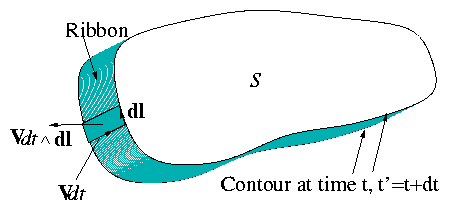Figure 4.16: Motion of contour with fluid gives convective flux derivative term.
Total rate of change of Φ is given by two terms:

 ⋅ Φ
 =

S

 ∂B ∂t . ds

Due to changes in B

+

 ⌠(⎜)⌡ C B. ( V ∧d l )

Due to motion of C

(4.168)
 =
 − ⌠⌡ S ∇ ∧E. ds − ⌠(⎜)⌡ C ( V ∧B) . d l
(4.169)
 =
 − ⌠(⎜)⌡ C (E+ V ∧B) . d l = 0    by  Ideal  Ohm′s  Law.
(4.170)
Flux through any surface moving with fluid is conserved.

## 4.13  Field Line Motion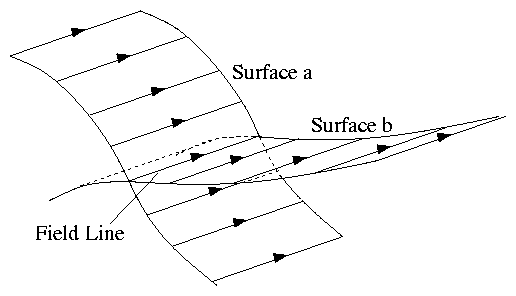Figure 4.17: Field line defined by intersection of two flux surfaces tangential to field.
Think of a field line as the intersection of two surfaces both tangential to the field everywhere:
Let surfaces move with fluid.
Since all parts of surfaces had zero flux crossing at start, they also have zero after, (by flux conservation).
That means their surfaces are tangent also after motion. Hence their intersection defines a field line after the motion.
We think of the new field line as the same line as the old one (only moved).
Thus:
1. Number of field lines ( ≡ flux) through any surface is constant. (Flux Cons.)
2. A line of fluid that starts as a field line remains one. Frozen-in field lines.

## 4.14  MHD Stability

The fact that one can find an MHD equilibrium (e.g. z-pinch) does not guarantee a useful confinement scheme because the equil. might be unstable. Ball on hill analogies: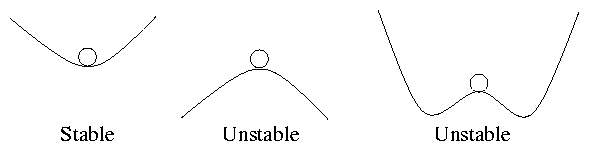Figure 4.18: Potential energy curves
An equilibrium is unstable if the curvature of the `Potential energy surface' is downward away from equil. That is if [( d2)/(dx2)] { Wpot } < 0.
In MHD the potential energy is Magnetic + Kinetic Pressure (usually mostly magnetic).
If we can find any type of perturbation which lowers the potential energy then the equil is unstable. It will not remain but will rapidly be lost.
Example Z-pinch
We know that there is an equilibrium: Is it stable?
Consider a perturbation thus: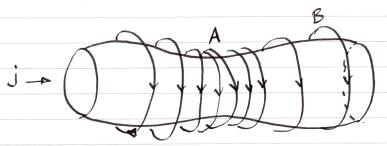Figure 4.19: 'Sausage' instability
Simplify the picture by taking the current all to flow in a skin. We know that the pressure is supported by the combination of B2/2 μo pressure and [(B2)/(μor)] tension forces.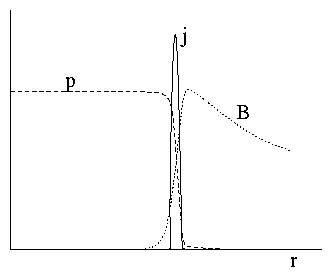Figure 4.20: Skin-current, sharp boundary pinch.
At the place where it pinches in (A)
Bθ and 1/r increase ⇒ Magnetic pressure & tension increase, ⇒ inward force no longer balanced by p, ⇒ perturbation grows.
At place where it bulges out (B)
Bθ & 1/r decrease ⇒ Magnetic pressure & tension decrease ⇒ perturbation grows.
Conclusion a small perturbation induces a small force imbalance tending to increase the perturbation. That means it is Unstable ( ≡ δW < 0).

## 4.15  General Perturbations of Cylindrical Equil.

Look for things which go like exp[i(kz + mθ)]. [Fourier (Normal Mode) Analysis].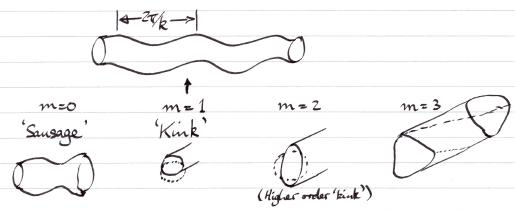Figure 4.21: Types of kink perturbation.
Generally Helical in form (like a screw thread). Example: m=1    k ≠ 0    z-pinch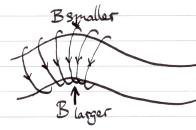Figure 4.22: Driving force of a kink. Net force tends to increase perturbation. Unstable.

## 4.16  General Principles Governing Instabilities

(1) They try not to bend field lines. (Because bending takes energy; think of a stretched elastic string). Not bending field lines requires perturbation (Constant surfaces) to lie along magnetic field.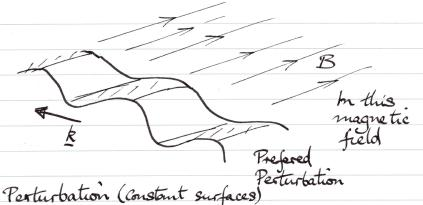Figure 4.23: Alignment of perturbation and field line minimizes bending energy.
Example: θ-pinch type plasma column: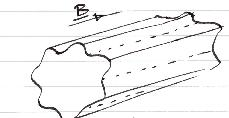Figure 4.24: `Flute' or `Interchange' modes.
Preferred Perturbations are `Flutes' as per Greek columns → `Flute Instability.' [Better name: `Interchange Instability', arises from idea that plasma and vacuum change places.]
(2)  Occur when a `heavier' fluid is supported by a `lighter' (Gravitational analogy). See Fig. 4.25.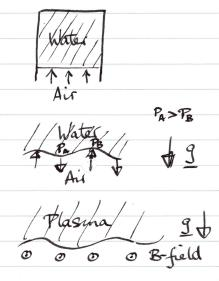Figure 4.25: Inverted water glass analogy. Rayleigh Taylor instability.
Why does water fall out of an inverted glass? Air pressure could sustain it but does not because of Rayleigh-Taylor instability.
Similar for supporting a plasma by mag field.
(3)  Occur when | B | decreases away from the plasma region. See Fig. 4.26.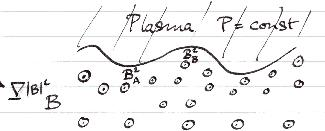Figure 4.26: Vertical upward field gradient is unstable.

 BA2 2 μo < BB2 2 μo
(4.171)
⇒ Perturbation Grows.
(4)  Occur when field line curvature is towards the plasma (Equivalent to (3) because of ∇ ∧B = 0 in a vacuum).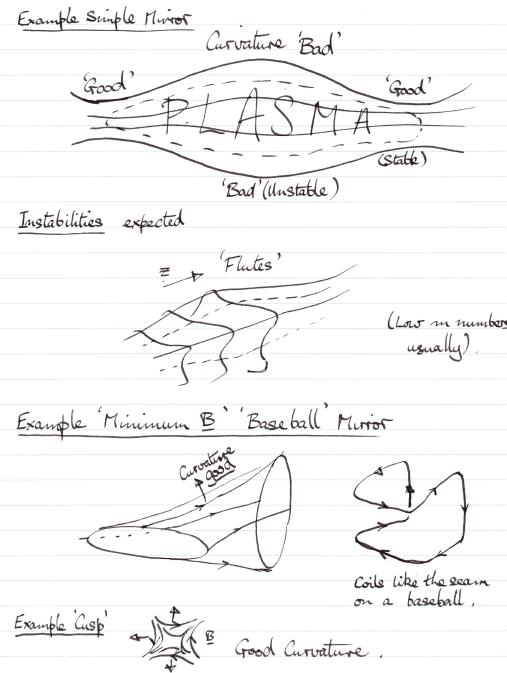Figure 4.27: Examples of magnetic configurations with good and bad curvature.

## 4.17  Quick and Simple Analysis of Pinches

θ-pinch      | B | = const. outside pinch
≡ No field line curvature.    Neutral stability
z-pinch     ∇ | B | away from plasma outside
≡ Bad Curvature (Towards plasma) ⇒ Instability.
Generally it is difficult to get the curvature to be good everywhere. Often it is sufficient to make it good on average on a field line. This is referred to as `Average Minimum B'. Tokamak has this.
General idea is that if field line is only in bad curvature over part of its length then to perturb in that region and not in the good region requires field line bending: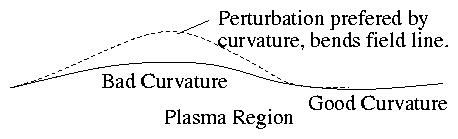Figure 4.28: Parallel localization of perturbation requires bending.
But bending is not preferred. So this may stabilize.
Possible way to stabilize configuration with bad curvature: Shear
Shear of Field Lines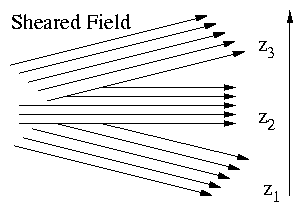Figure 4.29: Depiction of field shear.
Direction of B changes. A perturbation along B at z3 is not along B at z2 or z1 so it would have to bend field there → Stabilizing effect.
General Principle: Field line bending is stabilizing.
Example: Stabilized z-pinch
Perturbations (e.g. sausage or kink) bend Bz so the tension in Bz acts as a restoring force to prevent instability. If wave length very long bending is less. ⇒ Least stable tends to be longest wave length.
Example: `Cylindrical Tokamak'
Tokamak is in some ways like a periodic cylindrical stabilized pinch. Longest allowable wave length = 1 turn round torus the long way, i.e.
 kR = 1:      λ = 2 πR .
(4.172)
Express this in terms of a toroidal mode number, n (s.t. perturbation ∝ expi (nϕ+ mθ): ϕ = z/R    n = kR.
Most unstable mode tends to be n=1.
[Careful! Tokamak has important toroidal effects and some modes can be localized in the bad curvature region (n ≠ 1).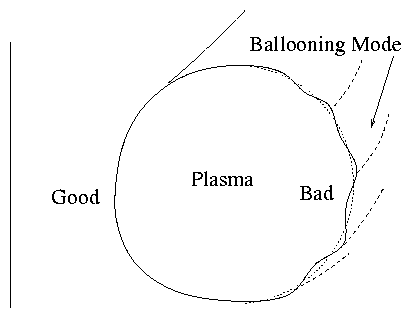Figure 4.30: Ballooning modes are localized in the outboard, bad curvature region.

## 4.18  Rayleigh Taylor Instability Analysis

Here we give an elementary mathematic derivation of the (in)stability criterion for a fluid supported against gravity and show that it is equivalent to a plasma under the influence of curvature forces. Although the treatment is general, rigor is sacrificed in the interests of brevity and clarity,
Consider a simple fluid (air, water, whatever). Its momentum equation is
 ρ ⎛⎝ ∂ ∂t + v.∇ ⎞⎠ v = −∇p + ρg,
(4.173)
where g is the gravity force vector pointing in the -z-direction. If the fluid starts at rest, then the term v.∇v is second order and ignorable in the linearized analysis we do.
We take the curl of the momentum equation (to annihilate ∇p) and get
 ∇∧ρ ∂v ∂t =∇∧ρg ( = ∇ρ∧g)
(4.174)
We write the equilibrium quantities as e.g. ρ0, and look for first-order perturbed quantities (e.g. ρ1) that vary like exp(ik.x), where k is perpendicular to z. One can show that the most unstable motions are displacements ξ that are in the vertical z-direction, so that k.v =0 and the flow is incompressible. Obviously, also v =∂ξ/∂t. The first order terms of eq. (4.174) are then
 ∇∧ ⎛⎝ ρ0 ∂2 ξ ∂t2 − gρ1 ⎞⎠ =0.
(4.175)
Using ξ's incompressibility in the continuity equation we find,
 ρ1=−ξ.∇ρ0 = − ξ ∂ρ0 ∂z ,
(4.176)
and when substituted into eq. (4.175) we get
 ∇∧ ⎛⎝ ρ0 ∂2 ξ ∂t2 + ξ ∂ρ0 ∂z g ⎞⎠ =0.
(4.177)
This equation is satisfied (only) by setting the bracket equal to zero, and noting that since its vectors (ξ =ξz, g =−gz) both point in the z direction it is effectively a scalar equation
 ∂2 ξ ∂t2 = ⎛⎝ g ρ0 ∂ρ0 ∂z ⎞⎠ ξ.
(4.178)
The solutions of this equation grow exponentially (unstably), with growth rate γ = √{[g/(ρ0)][(∂ρ0)/(∂z)]}, if ([g/(ρ0)][(∂ρ0)/(∂z)]) > 0. They are instead are purely oscillatory (stable) if ([g/(ρ0)][(∂ρ0)/(∂z)]) < 0. The criterion for instability, which can be written more formally as g.∇ρ0 < 0, can be thought of informally as saying "a lower density (lighter) fluid supported by a higher density (heavier) fluid is unstable". All of this is for neutral fluids, the instability is called Rayleigh-Taylor.

### MHD plasma stability. Magnetic Field with gravity

The momentum equation can be written
 ρ ⎛⎝ ∂ ∂t + v.∇ ⎞⎠ v = −∇ ⎛⎝ p + B2 2μ0 ⎞⎠ + 1 μ0 (B.∇)B+ ρg,
(4.179)
If the term (B.∇)B were ignorable (and the directions of g and ∇ρ0 coincide and are perpendicular to B) the analysis would be identical. That term (B.∇)B has two possible first order parts: (B0.k)B1 and (B1.∇)B0, I will ignore the second and concentrate only on the first. It is zero if B0.k =0, that is, if the perturbation wave-fronts are field-aligned. Nothing then stabilizes the Rayleigh-Taylor instability if g.∇ρ0 < 0. If the term B0.kB1 is not zero, then
 ∇∧ ⎡⎣ ρ0 ∂2 ξ ∂t2 + ξg.∇ρ0 −(B0.ik)B1/μ0 ⎤⎦ =0.
(4.180)
The g-component (formerly the z-component) of B1 must be considered. By the freezing in of field-lines, and simple geometry,
 B1g = B0.∇ξg = i(B0.k)ξg.
(4.181)
Therefore the scalar equation that must be satisfied is
 − ∂2 ξ ∂t2 = 1 ρ0 [g.∇ρ0+(B0.k)2/μ0]ξ.
(4.182)
The magnetic term is always positive, which is stabilizing. If (B0.k)2 > −g.∇(ρ0), then the mode is stable (oscillatory). Otherwise the destabilizing g-term overcomes the stabilizing bending term.

### Equivalence of Field-Curvature and Gravity

In a vacuum field, the combined grad-B and curvature drift is
 vd=(mv⊥2/2 + mv||2) Rc∧B qRc2B2 ,
(4.183)
which can be considered to arise from the generalized force expression with the centrifugal and −μ∇B forces. By simple inspection of the above equation, the force per particle giving rise to it is (mv2/2 + mv||2)Rc/Rc2. Averaged over a Maxwellian distribution, both the energy terms are T, so the force density is
 Fc = 2nTRc/Rc2 = 2pRc/Rc2.
(4.184)
This is the quantity that is equivalent to ρ0g. It points in the direction of the radius of curvature Rc. If ∇.Fc < 0, then the plasma is unstable to perturbations for which k.B0=0. The more general instability criterion for non-field-aligned perturbations is
 (Rc.∇p)/Rc2 < (B0.k)2/2μ0.
(4.185)
Then we think of ∇p as being "inward", toward the hot dense center of a confined plasma. In which case the radius curvature being "outward" is unfavorable for stability.
[It might seem that since we always need the most unstable mode, the stabilizing effects of B0.k are irrelevant because the most unstable mode will always choose this to be zero. However, if field has shear then as soon as one moves away (in the g direction) from the place where B0.k =0 has been chosen, the field angle changes; so B0.k is no longer zero. Consequently, there will be a trade-off between localization of the eigenmode in the g-direction which implies compression, and field line bending. Also, sometimes Fc can vary from stabilizing to destabilizing along the field; then no perturbation that zeroes B0.k everywhere can be localized to where Fc is destabilizing.]